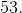# SSAT Elementary Level Math : How to find the part from the whole

## Example Questions

### Example Question #32 : Number & Operations In Base Ten

What digit is in the ones place?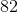NeitherExplanation:

The ones place is always the first number on the right.

### Example Question #1 : Numbers 10, 20, 30, 40, 50, 60, 70, 80, 90 Refer To One, Two, Three, Four, Five, Six, Seven, Eight, Or Nine Tens: Ccss.Math.Content.1.Nbt.B.2.C

How many tens are in the number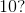Ten

Six

Five

One

Two

One

Explanation:

There is ain the tens place which means there is one ten in the number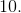### Example Question #71 : How To Find The Part From The Whole

How many tens are in the number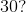Thirteen

Five

Thirty

Four

Three

Three

Explanation:

There is ain the tens place which means there are three tens in the number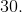### Example Question #2 : Numbers 10, 20, 30, 40, 50, 60, 70, 80, 90 Refer To One, Two, Three, Four, Five, Six, Seven, Eight, Or Nine Tens: Ccss.Math.Content.1.Nbt.B.2.C

How many tens are in the number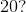Twelve

Three

Two

Twenty

One

Two

Explanation:

There is ain the tens place which means there are two tens in the number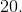### Example Question #1 : Numbers 10, 20, 30, 40, 50, 60, 70, 80, 90 Refer To One, Two, Three, Four, Five, Six, Seven, Eight, Or Nine Tens: Ccss.Math.Content.1.Nbt.B.2.C

How many tens are in the number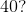Five

Four

Six

Fourteen

Forty

Four

Explanation:

There is ain the tens place which means there are four tens in the number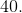### Example Question #2 : Numbers 10, 20, 30, 40, 50, 60, 70, 80, 90 Refer To One, Two, Three, Four, Five, Six, Seven, Eight, Or Nine Tens: Ccss.Math.Content.1.Nbt.B.2.C

How many tens are in the number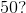Three

Fifty

Five

Six

Seven

Five

Explanation:

There is ain the tens place which means there are five tens in the number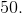### Example Question #3 : Numbers 10, 20, 30, 40, 50, 60, 70, 80, 90 Refer To One, Two, Three, Four, Five, Six, Seven, Eight, Or Nine Tens: Ccss.Math.Content.1.Nbt.B.2.C

How many tens are in the number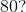Eight

Four

Sixty

Six

Eighty

Eight

Explanation:

There is ain the tens place which means there are eight tens in the number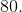### Example Question #1 : Numbers 10, 20, 30, 40, 50, 60, 70, 80, 90 Refer To One, Two, Three, Four, Five, Six, Seven, Eight, Or Nine Tens: Ccss.Math.Content.1.Nbt.B.2.C

How many tens are in the number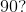Nine

Ninty

Four

Three

Forty

Nine

Explanation:

There is ain the tens place which means there are nine tens in the number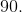### Example Question #8 : Numbers 10, 20, 30, 40, 50, 60, 70, 80, 90 Refer To One, Two, Three, Four, Five, Six, Seven, Eight, Or Nine Tens: Ccss.Math.Content.1.Nbt.B.2.C

How many tens are in the number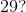Two

Twenty

Three

Nine

Twenty-nine

Two

Explanation:

There is ain the tens place which means there are two tens in the number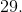### Example Question #9 : Numbers 10, 20, 30, 40, 50, 60, 70, 80, 90 Refer To One, Two, Three, Four, Five, Six, Seven, Eight, Or Nine Tens: Ccss.Math.Content.1.Nbt.B.2.C

How many tens are in the number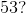Fifty

Thirty

Three

Five

Fifty-three

There is ain the tens place which means there are five tens in the number